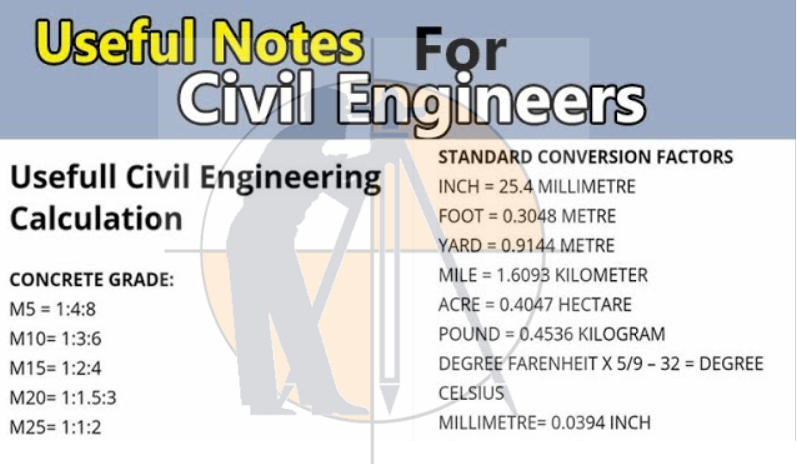Breaking News
Home / Civil Engineering / Civil Engineering Measurements And Conversion Factor

# Civil Engineering Measurements And Conversion Factor

## Civil Engineering Measurements And Conversion Factors

Estimation is a standout among the most imperative things in structural building and without estimations, we can not finish any development. Here I have recorded some essential estimations and change factors which are most generally utilized as a part of structural designing. Expectation it will help you in your work.Basic Conversions a Civil Engineer on Site Must Learn basic must be learn.A unit of measurement is a defined magnitude of a quantity that it used as a standard for measurement for the same kind of quantity, such as measurements of length, weight, and volume.

• 1 bag of cement = .0347 cum
• 1 sqm = 10.76 sqft
• 1 inch = 25.4 mm
• Weight in Kilograms of Mild Steel (Sheet,grill etc.) = (Volume x Density of Mild Steel) = Length x Width x Thickness x 7850 kg/cum. Eg. 2.5 m x 1.25 m x .001 m x 7850 = 24.53 KGs……….. [ Keep a notice on the units ]
• 1 tonne = 1000 KGs
• 1 quintal = 100 KGs
• 1 Acre = 43560 sq. ft = 4046.46 sqm
• 1 cum = 35.32 cuft
• Unit Weight of Steel Bar = D^2/162 where D is the diameter of the bar. Eg. 12 mm dia bars, unit weight = 12*12/162 = 0.89 kg/m. [Unit weight in this case is the weight of steel bar per meter ].
• 1 ton and 1 tonne are different things. 1 ton is US ton which is 907.18 KGs. 1 tonne or 1 MT(usually referred as MT in BOQs) is 1000 KGs.
• In some cases earthworks will be in % Cum wherein you will need to divide the amount by 100. Eg. Earth work in excavation of foundation trenches or drains, Qty = 24295 %cum Rate = Rs. 9161.6 Amount = 24295*9161.6/100.

## Civil Engineering Measurements & Conversion Factors

#### BASIC QUANTITIES AND UNITS:

 Quantity Dimension SI Unit FPS Unit Mass M Kg Slug Length L M ft. Time T sec. sec. Force F Newton (N) Pound (lb)
BASIC STANDARDS:
1 meter = 39.37 inches =1.09 yards.
1 inch = 25.4 millimeters = 2.54cm.
1 Kilogram (kg) = 2.2046 pounds (lb).
1 gallon (imp.) = 4.546 liters.
1 liter = 0.22 galls (imp.)
1 gallon (US) = 3.785 liters.
________________________________________
METRIC UNIT OF WEIGHT/MASS:
1 tonne = 1000 kilograms = 1,000,000 grams.
1 quintal = 100 kilograms = 100,000 grams.
1 Slug = 14.606 kg
1 Slug = 32.2 lb
________________________________________
MEASUREMENTS OF LENGTH:
1 foot = 12 inches.
1 yard = 3 feet.
1 furlong = 220 yards.
1 mile = 8 fulongs.
1 Kilometer (Km) = 1000 meters.
1 meter (m) = 100 centimeters (cm).
1 cm = 10 millimeter (mm).
________________________________________
METRIC UNITS FOR LIQUID MEASUREMENTS:
1 liter = 1000ml.
1 liter = 1kg.
1 kiloliter (1000 liters) = 1 cubic meter/ 1 cu.m/ 1 m3.
________________________________________
CONVERSION FACTORS:
1 cu. ft. = 28.317 liters.
1 cu. ft. = 0.028 cu. meters.
1 cu. ft. = 6.24 galls (imperial).
1 cu. ft. = 7.48 galls (US).
1 imp. gall = 1.20 galls (US), liquid.
1 imp. gall = 1.03 galls (US), dry.

### How To Find The Coordinates Of  Point P With The Help Of Point A & B

________________________________________
MEASUREMENTS OF AREA:
1 Acre = 43560 sq. ft
1 Acre = 4046.46 sq. m
1 Acre = 8 Kanals.
1 Kanal = 20 Marlas.
1 Marla = 225 sq. ft (* in some regions 272 sq. ft)
1 Marla = 15.50 sq.m
________________________________________
MISCELLENIUS CONVERSION FACTORS:
1 cu.m = 35.32 cu.ft.
1 Pound = 4.448 Newton (Force).
1 klb = 4.448 kN.
1 Psi (lb/sq.in) = 6.689 Pascal (N/sq.m)
1 (lb/sq. ft) = 0.048 (kN/sq.m)

# Convert USCS into SI Units

 USCS unit X Factor = SI unit SI symbol Square foot X 0.0929 = Square meter M2 Cubic foot  X 0.2831 = Cubic meter M3 Pound per square inch X 6.894 = Kilopascal KPa Pound force X 4.448 =  Newton Nu Foot pound torque X 1.356 = Newton meter N-m Kip foot X 1.355 = Kilo-newton meter LN-m Gallon per minute X 0.06309 = Liter per second L/s Kip per square inch X 6.89 = Mega-pascal MPa

Basic Conversions a Civil Engineer on Site Must Learn useful for civil engineer in field work.

Get Benefits & Share With Other.

### THANKS.